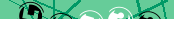# JavaScript: Using objects as array keys

In setting up our Amazing Maze Game we created an object Position{x, y} to store the position of the hero in the maze, and a 2-dimensional array maze[x][y] representing the maze grid, but this led to some awkward coding which would be simplified if our Position object could be used as an array index.

## Defining the problem

Consider the following simple JavaScript object:

```function Position(x, y) { this.x = x; this.y = y; }```

We can now assign a position to our hero using:

`var heroPos = new Position(2, 2);`

and if we define our maze grid as:

```/* [1, 2, 3], [4, 5, 6], [7, 8, 9] /* var maze = []; maze = 1; meze = 2; meze = 3; meze = 4; ... maze = 9; // <-- heroPos```

this places the hero in the bottom right corner where the value is nine.

Only to actually get the value of that grid position in the maze we have to use:

`maze[heroPos.x][heroPos.y]; // maze == 9`

What we'd like to be able to use instead is:

`maze[heroPos]; // maze[Position{2,2}] == 9`

## Why can't objects be keys

To set up our maze using Position objects as keys we use something like the following:

```var maze = []; maze[new Position(0, 0)] = 1; maze[new Position(0, 1)] = 2; maze[new Position(0, 2)] = 3; maze[new Position(1, 0)] = 4; ... maze[new Position(2, 2)] = 9; // <-- heroPos```

But this doesn't work as expected as we end up with only a single array value:

```for(var x in maze) { console.log(x + " => " + maze[x]); } [object Object] => 9```

What's happening? It turns out that when you try to use an object as an array key the objects toString() method is invoked, which by default returns the string "[object Object]" regardless of the object attribute values.

## How to make it work

It turns out there's a very simple solution. Knowing that the objects toString() method is going to be invoked we just have to overwrite it with our own:

```function Position(x, y) { this.x = x; this.y = y; } Position.prototype.toString = function() { return this.x + ":" + this.y; };```

Now when we populate our maze as before, instead of the "[object Object]" we see our new custom values for the whole 3×3 grid:

```for(var x in maze) { console.log(x + " => " + maze[x]); } 0:0 => 1 0:1 => 2 0:2 => 3 1:0 => 4 ... 2:2 => 9```

And we can now use the desired syntax:

`maze[heroPos]; // maze["2:2"] == 9`

To see the code in action check out our Amazing maze game with source code.

## Why Map didn't work

The little-known Map object could potentially have saved us from having to add the toString prototype as it is essentially an array which supports objects as keys:

``` function Position(x, y) { this.x = x; this.y = y; } var mazeMap = new Map(); var pos = new Position(1, 1); mazeMap.set(pos, "here I am!"); console.log(mazeMap.get(pos)); // here I am!```

But it only works for the exact same object (===) and not just an object with identical values (==):

``` console.log(mazeMap.get(new Position(1, 1))); // undefined```

Ironically, it will work if we convert the object to a string using our toString prototype:

``` var pos = new Position(1, 1); mazeMap.set(pos.toString(), "here I am!"); console.log(mazeMap.get(pos.toString())); // here I am! console.log(mazeMap.get(new Position(1, 1).toString())); // here I am!```

< JavaScript

#### Send a message to The Art of Web: